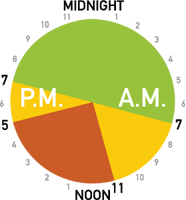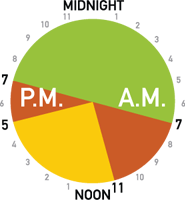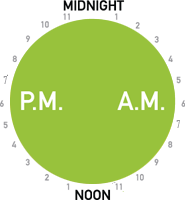# Plug it back in tonight or this weekend

This is my first code golf so please let me know if it's too broad or if I'm missing any information for a good puzzle!

# Challenge

In Ontario and possibly other areas of the world, electricity is billed using Time-Of-Use (TOU) pricing, which varies the cost per kilowatt-hour according to when you use power.

Given a date and time, I want to know whether I'm in an on-peak (red), mid-peak (yellow), or off-peak (green) time period.

# Input

Assume that input is provided in an acceptable timezone-less ISO 8601 date-time format with the minimum precision of hours: YYYY-MM-DDThh[:mm[:ss]] (the T is literal).

### Examples

• 2014-09-01T14
• 2014-09-01T17:30
• 2014-09-01T17:30:02

# Output

The output should be a string On, Mid, or Off.

# Rules

• Shortest code wins
• For the purposes of this challenge, ignore statutory holidays
• Assume the information found in this post. The actual rules of time-of-use pricing might change in the future by the Ontario Ministry of Energy.

# Information

## Summer weekdays (May 1st to October 31st)• Off-peak: 19h00 - 07h00
• Mid-peak: 07h00 - 11h00 and 17h00 - 19h00
• On-peak: 11h00 - 17h00

## Winter weekdays (November 1st to April 30th)• Off-peak: 19h00 - 07h00
• Mid-peak: 11h00 - 17h00
• On-peak: 07h00 - 11h00 and 17h00 - 19h00

## Weekends• Off-peak: All day
• Are you sure the winter weekdays don't have mid-peak / on-peak swapped? Aug 26, 2014 at 8:52
• @JanDvorak, in winter people use lights and heating in the morning and evening; in summer they use air-con at midday. Aug 26, 2014 at 8:53
• This is borderline-duplicate of codegolf.stackexchange.com/q/7008/194 (parse a datetime and do a simple calculation based on whether it's a working day or not). I think the season dependency is just different enough, but others may disagree. Aug 26, 2014 at 8:55
• @PeterTaylor the rules seem much simpler here than in the linked question. This doesn't have to handle leap years, for example. Aug 26, 2014 at 9:00
• Should the general date format be YYYY-MM-DDThh[:mm[:ss]] since seconds can only be applied if minutes are applied? Aug 26, 2014 at 14:34

# Python 2 - 164

from datetime import*
d,t=input().split('T')
y,m,d=map(int,d.split('-'))
t=int(t[:2])
print'OMOfinfd'[(1+((10<t<17)==(4<m<11)))*(date(y,m,d).weekday()<5<6<t<19)::3]


If needed, below is a explanation of the logic in the final line:

The final line prints a slice of 'OMOfinfd' depending on the evaluation of its conditionals.

• First, evaluate the operation 1+((10<t<17)==(4<m<11)).

If the XNOR between the conditions 10<t<17 and 4<m<11 is False, this will evaluate to 1+False => 1+0 => 1. Otherwise, the operation will evaluate to 1+True => 1+1 => 2.

• Finally, multiply that result of the above operation by whether the day is a weekday and whether the time is between 6am-7pm.

If this is False, either the day is a weekend or the time is between 7pm-6am, and the result will be (1|2)*0 => 0. Otherwise the result will be (1|2)*1 => 1|2.

A result of 0 will print Off, 1 will print Mid, and 2 will print On.

• Isn't the golfed version actually longer because you use semicolons? Or are newlines counted? Aug 26, 2014 at 14:59
• Newlines are typically counted. In Notepad++ (where I typically get my counts) the ungolfed version is 4bytes longer at 168. Aug 26, 2014 at 15:54
• @BeetDemGuise Edit → EOL Conversion → UNIX/OSX Format. Aug 26, 2014 at 16:20

## C# - 240 220 chars

string x(string s){var d=DateTime.Parse((s+":00:00").Substring(0,19));int h=d.Hour,i=(int)d.DayOfWeek,x=d.Month;string o="off",m="mid",f="on";return i==6|i==0?o:x>=5&x<11?h>18|h<7?o:h>10&h<17?f:m:h>18|h<7?o:h>10&h<17?m:f;}


Nothing special. Straight forward coding.

Thanks to w0lf :)

• I think you can shorten s.Length==13?s+":00:00":s.Length==16?s+":00":s to (s+":00:00").Substring(0,19) Aug 26, 2014 at 15:41

## Ruby - 135

Abuses the Time module. Input by command line argument.

d=Time.new(*$*.scan(/\d+/)[0..3]) o,m,f=%w{On Mid Off} o,m=m,o if (d.mon-5)%10<6 p d.wday%6<1||(12>h=(d.hour+5)%24)?f:15<h&&h<22?m:o  Edit: Thanks w0lf for Time which helped shorten and solve a bug. • Your program is incorrect. For the input 2014-09-01T17:30 it correctly outputs "Mid", but for 2014-09-01T17 it outputs "Off". Aug 28, 2014 at 13:51 ## Ruby - 147 144 143 141 137 135 x=->s{y,m,d,h=s.scan(/\d+/).map &:to_i g=Time.new(y,m,d).wday%6<1?0:[0..11,4..9].count{|r|r===h-7} %W{Off Mid On}[m<5||m>10?(3-g)%3:g]}  This represents a function which takes a string as a parameter and returns a string. Here's an online demo with some test cases: http://ideone.com/wyIydw ## Groovy - 621534524 491 chars Some further golfing to do, but pretty simple when leveraging Joda-Time @Grab(group='joda-time',module='joda-time',version='2.3') f={p,a->org.joda.time.format.DateTimeFormat.forPattern(p).parseDateTime a} g={p,a->def x=null;try{x=f p,a}catch(Exception e){}} a=args d=["",":mm",":mm:ss"].collect{g "yyyy-MM-dd'T'HH$it",a}.find{it}
r="Off"
m="Mid"
j={d,a,b->d.hourOfDay>a&&d.hourOfDay<b}
k={j(it,6,11)||(j(it,16,19))}
if(d.dayOfWeek<6){x=d.monthOfYear;if(x>4&&x<12){if(j(d,10,17))r="On";if(k(d))r=m}else if(x<5||x>10){if(j(d,10,17))r=m;if(k(d))r="On"}}
println r


sample runs:

bash-3.2$./run.peak.sh groovy Peak.groovy 2014-08-26T19 Off groovy Peak.groovy 2014-08-26T07:00 Mid groovy Peak.groovy 2014-08-26T18:00:00 Mid groovy Peak.groovy 2014-08-26T12:30:30 On groovy Peak.groovy 2014-11-01T00 Off groovy Peak.groovy 2014-02-05T11:11:11 Mid groovy Peak.groovy 2014-01-05T08:08 Off groovy Peak.groovy 2014-12-18T18:59:59 On groovy Peak.groovy 2014-08-31T14 Off  Ungolfed: @Grab(group='joda-time',module='joda-time',version='2.3') f = { p,a -> org.joda.time.format.DateTimeFormat.forPattern(p).parseDateTime a} g = { p,a -> def x=null; try{x=f p,a} catch(Exception e) {} } a=args d = ["",":mm",":mm:ss"].collect{g "yyyy-MM-dd'T'HH$it",a}.find{it}
r = "Off"
m = "Mid"

j = {d,a,b -> d.hourOfDay > a && d.hourOfDay < b}
k = { j(it,6,11) || (j(it,16,19)) }

if (d.dayOfWeek<6) {
x = d.monthOfYear;
if ( x>4 && x<12 ) {
if (j(d,10,17)) r="On";
if (k(d)) r=m
} else if (x<5||x>10) {
if (j(d,10,17)) r=m;
if (k(d)) r="On"
}
}
println r

• Since Java 8, there's also java.time.*, which is very similar to Joda Time but is part of the JRE. Perhaps this could shorten the code a bit. Aug 26, 2014 at 11:37
• re: Java 8. excellent point Aug 26, 2014 at 12:15
• In your 3rd-to-last example '2014-01-05T08:08', January 5th, 2014 is a Sunday. Thus, it should be 'Off' Aug 26, 2014 at 13:56
• re: Jan 5, 2014. Quite right. The current code is correct, but the output run is wrong. Will fix. Aug 26, 2014 at 14:18
• Tried java.time.*. failed miserably. The parser is too damn strict. Use DateParser from nashorn (also part of the JRE8) which is lenient, and with a bit of abusive hacking, even lenient enough to skip minutes&seconds. Aug 27, 2014 at 19:40

## R, 243 204 characters

b=strptime(scan(,""),"%Y-%m-%dT%H");i=function(x)as.integer(format(b,x));h=i("%H");d=i("%m%d");w=d>1100|d<430;f=ifelse;cat(c("Off","Mid","On")[f(i("%u")%in%5:6|h<7|h>19,1,f(h>11&h<17,f(w,2,3),f(w,3,2)))])


Indented and commented:

b=strptime(scan(,""),"%Y-%m-%dT%H") #Takes stdin and converts into POSIXct
i=function(x)as.integer(format(b,x)) #Format the POSIXct and convert it to integer
h=i("%H")      #Format to hours
d=i("%m%d")    #Format to Month/Day
w=d>1100|d<430 #True if winter time, false if summer
f=ifelse
cat(c("Off","Mid","On")[f(i("%u")%in%5:6|h<7|h>19, #If weekend or night
1,                       #Case 1
f(h>11&h<17,            #Else if mid-day
f(w,3,2),             #Case 2 in winter, case 3 in summer
f(w,2,3)))])          #else vice versa


Examples:

> b=strptime(scan(,""),"%Y-%m-%dT%H");i=function(x)as.integer(format(b,x));h=i("%H");d=i("%m%d");w=d>1100|d<430;f=ifelse;cat(c("Off","Mid","On")[f(i("%u")%in%5:6|h<7|h>19,1,f(h>11&h<17,f(w,3,2),f(w,2,3)))])
1: 2014-08-26T15
2:
On
> b=strptime(scan(,""),"%Y-%m-%dT%H");i=function(x)as.integer(format(b,x));h=i("%H");d=i("%m%d");w=d>1100|d<430;f=ifelse;cat(c("Off","Mid","On")[f(i("%u")%in%5:6|h<7|h>19,1,f(h>11&h<17,f(w,3,2),f(w,2,3)))])
1: 2014-12-10T15
2:
Mid
> b=strptime(scan(,""),"%Y-%m-%dT%H");i=function(x)as.integer(format(b,x));h=i("%H");d=i("%m%d");w=d>1100|d<430;f=ifelse;cat(c("Off","Mid","On")[f(i("%u")%in%5:6|h<7|h>19,1,f(h>11&h<17,f(w,3,2),f(w,2,3)))])
1: 2014-08-26T23
2:
Off


## Bash, 286

this is a simple bash answer using the date program

d(){ date -d $1 +%$2; };D=$(echo$1|sed 's/$$T..$$$/\1:00/');H=$(d $D H);M=$(d $D m);if [$(d $D u) -gt 5 ]||[$H -lt 7 ]||[ $H -gt 18 ];then echo Off;exit;fi;if [$M -gt 4 ]&&[ $M -lt 11 ];then I=On;O=Mid;else I=Mid;O=On;fi;if [$H -gt 10 ]&&[ $H -lt 17 ];then echo$I;else echo $O;fi  Here goes another one! # JavaScript, 175 171 function f(x){d=new Date(x.slice(0,10));t=x.slice(11,13),m=(x.slice(5,7)+1)%12;return(t<8||t>18||!(d.getUTCDay()%6)?'off':((t<11||t>17)?(m<5?'on':'mid'):(m<5?'mid':'on'))}  Unminified: function f(x) { d = new Date(x.slice(0, 10)); t = x.slice(11, 13), m = (x.slice(5, 7) + 1) % 12; return (t < 8 || t > 18 || !(d.getUTCDay() % 6) ? 'off' : ((t < 11 || t > 17) ? (m < 5 ? 'on' : 'mid') : (m < 5 ? 'mid' : 'on')) }  Only works on interpreters where an ISO8601 date string can be passed into the Date constructor. # CoffeeScript, 192 189 Surprisingly, it's longer in CoffeeScript because there's no ternary operator in that language (which as you can see from my JavaScript, I heavily relied on). f=(x)->d=new Date(x.slice(0,10));t=x.slice(11,13);m=(x.slice(5,7)+1)%12;return'off'if(t<8||t>18||!(d.getUTCDay()%6));if(t<11||t>17)then(if m<5then'on'else'mid')else(if m<5then'mid'else'on')  # ES6 - 146 This is in function form, uses a couple nasty hacks. let y,m,d,h,c=s=>[y,m,d,h]=s.split(/[T:-]/g).map(i=>+i),new Date(y,m,d).getDay()in{0:1,6:1}||h<20||8>h?'Off':['Mid','On'][(10>h&&h>16)^(m>5||m<8)]  Explained: // These variables are declared outside of the function scope to save // characters. let y, // year m, // month d, // day h, // hour c = s => // c for check, called as c(isoString) [y, m, d, h] = // Assign to fields s.split(/[T:-]/g) // Split at delimiters .map(i => +i), // Convert all to numbers // Comma used to keep it as a single statement. new Date(y, m, d).getDay() // Create Date to get day of week in {0:1, 6:1} // Check if it is good (0 = Sunday, 6 = Saturday). It // is shorter to use an object literal than to // do individual checks. || h < 20 || 8 > h ? // h is between 7 and 19 (7pm) 'Off' : // Return 'Off' ['Mid','On'][ // Two other outputs (10 > h && h > 16) ^ // Check if it is 11-16 (5pm) (m > 5 || m < 8)] // Invert that if the month is in summer/fall. Note // that this returns a number, either 1 or 0. This // is an ugly hack using the bitwise xor operator.  # Python 3 - 352 chars import datetime as dt t=input() i=int p=print a='on' b='mid' c='off' m=i(t[5:7]) h=i(t[11:13]) d=dt.date(i(t[0:4]),m,i(t[8:10])).weekday() if 5==d or 6==d:p(c) elif h>=19 and h<7:p(c) elif m<=10 and m>=4: if h>=7 and h<11:p(b) if h>=11 and h<17:p(a) if h>=17 and h<19:p(b) else: if h>=7 and h<11:p(a) if h>=11 and h<17:p(b) if h>=17 and h<19:p(a)  • You should change s=['high','mid','off'] to s=['on','mid','off'] - not only does this save 2 chars, but the spec says to output "on". Aug 26, 2014 at 12:27 ## Java - 426 309 / 301? (see comments) String z(String a){ DateParser b=new DateParser(a); boolean c=b.parseEcmaDate(); Integer[]d=b.getDateFields(); GregorianCalendar e=new GregorianCalendar(d,d-(c?0:1),d); e.setTimeZone(new SimpleTimeZone(0,"")); return(124>>e.get(7)&1)>0&d>6&d<19? d>10&d<17^(1008>>e.get(2)&1)>0?"Mid":"On":"Off"; }  Example output: 2014-03-02T00 Off 2014-03-02T06 Off 2014-03-02T07 Off 2014-03-02T10 Off 2014-03-02T11 Off 2014-03-02T16 Off 2014-03-02T17 Off 2014-03-02T18 Off 2014-03-02T19 Off 2014-03-02T23 Off 2014-04-02T00 Off 2014-04-02T06 Off 2014-04-02T07 On 2014-04-02T10 On 2014-04-02T11 Mid 2014-04-02T16 Mid 2014-04-02T17 On 2014-04-02T18 On 2014-04-02T19 Off 2014-04-02T23 Off 2014-05-02T00 Off 2014-05-02T06 Off 2014-05-02T07 Mid 2014-05-02T10 Mid 2014-05-02T11 On 2014-05-02T16 On 2014-05-02T17 Mid 2014-05-02T18 Mid 2014-05-02T19 Off 2014-05-02T23 Off  I used the same EXOR trick as the Python submission. I also used a + as an OR function, for when it's weekend OR night. My other big trick: bit masks. For example, to see if a number is between 2 and 6 (Monday to Friday), first create a bit pattern where the interesting values are 1:  6 2 0b1111100 = 124  Then, use bit shift to get the interesting bit to the LSB and extract it: (124 >> day_of_week) & 1  Similarly, I made bit patterns for months and hours:  9 4 0b1111110000 = 1008 76 76 0b111110000000000001111111 = 16253055 0b000000011111100000000000 = 129024  Unfortunately, it turns out simply x>y&x<z is shorter in most cases, so I didn't use it in some places. And finally, a bit of hackery (highly implementation dependent) with jdk.nashorn.internal.parser.DateParser: When parseEcmaDate() doesn't completely parse a date (like when it reads hour and hits end of string), it returns false. • When it finishes properly, the date is stored in an Integer[] (auto-unboxing ftw), with the month fixed to be base-0 (like other Java classes). • When it returns false, it aborted half-way and didn't do this fixing. It however still put whatever it parsed into the array, which is readily available. Hence the -(c?0:1). • I think using just .parse() might also work (shaving off 8 characters), but I haven't tested it thoroughly with different inputs. parse internally calls parseEcmaDate and if it fails, calls parseLegacyDate. The latter may screw up the array, but this didn't happen with a few cases I tested. Aug 27, 2014 at 20:48 Nothing stops me from entering my own, and there's other shorter ones here anyways, so… # PHP 5.4+, 194 <?function f($x){$t=substr($x,11,2);$m=(substr($x,5,2)+1)%12;if($t<8||$t>18||(new DateTime(substr($x,0,10)))->format('N')>5)return'off';return($t<11||$t>17)?($m<5?'on':'mid'):($m<5?'mid':'on');}  Unminified and commented: <? function f($x) {
$t = substr($x,11,2); // hour
$m = (substr($x,5,2) + 1) % 12; // month shifted up by 1

if ($t < 8 ||$t > 18 || (new DateTime(substr($x,0,10)))->format('N') > 5) return 'off'; // evenings and weekends return ($t < 11 || $t > 17) ? ($m < 5 ? 'on' : 'mid') // morning and mid-afternoon
: (\$m < 5 ? 'mid' : 'on'); // afternoon
}


Also note that the date.timezone directive in php.ini must be set, otherwise an Exception will be thrown.